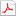검색어 입력폼

# Rational Numbers

저작시기 2012.05 | 등록일 2012.05.25어도비 PDF (pdf) | 5페이지 | 가격 2,000원

## 소개글

introduction to ratioal numbers: definition, structure, properties

없음

## 본문내용

Rational Numbers
Rational numbers are whole numbers, fractions, and decimals - the numbers we use in our daily lives. In mathematics, the formal definition of a rational number is any number that can be expressed as the quotient or fraction a/b of two integers, with the denominator b not equal to zero. Since b may be equal to 1, every integer is a rational number. In other words, a rational number is a number that can be written as a simple fraction or as a ratio. For example, 1.5 is a rational number because 1.5 = 3/2.
In contrast, a real number that is not rational is called irrational. Because the square root of 2 cannot be written as a simple fraction, it is not a rational number, which means an irrational number, and there are many more such numbers as π, and e.

없음